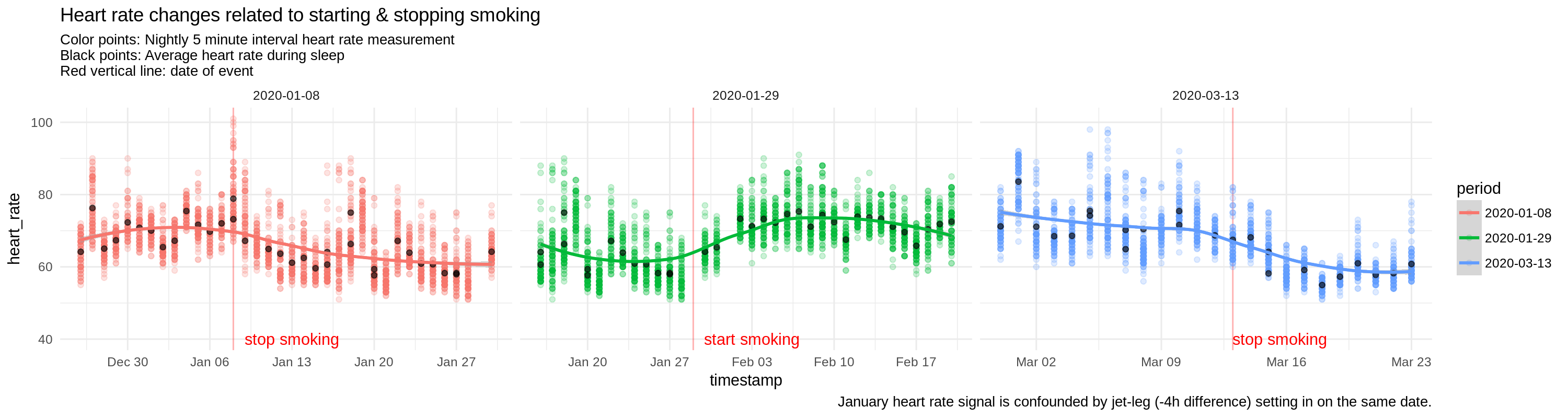# Details for `heart-rate-and-smoking.ipynb`

#### Description

How does the resting & nightly heart rate change when smoking

#### Tags & Data Sources

heart rate smoking Oura Connect

# Resting Heart rate after starting/stopping to smoke¶

This notebook takes some dates on which you started/stopped smoking and shows the heart rate before/after those events.

The code below will now get your data from Open Humans and create a table with all the data points in the date ranges you specified above:

### Heart rate changes¶

These graphs follow the same pattern, but as Oura records a heart rate value every 5 minutes during sleep, we'll see lots of points per night, giving us a pattern for each day. The red, vertical line again shows the day of the incident and the fit-line tries to show how the points distribute for each day.

```/opt/conda/lib/python3.6/site-packages/rpy2/robjects/pandas2ri.py:57: UserWarning: Error while trying to convert the column "timestamp". Fall back to string conversion. The error is: Conversion 'py2ri' not defined for objects of type '<class 'arrow.arrow.Arrow'>'
(name, str(e)))
```# Resting Heart rate after starting/stopping to smoke¶

This notebook takes some dates on which you started/stopped smoking and shows the heart rate before/after those events.

In :
```events = ['2020-01-08', '2020-01-29', '2020-03-13']
weeks_before_event = 2
weeks_after_event = 3
```

The code below will now get your data from Open Humans and create a table with all the data points in the date ranges you specified above:

In :
```from ohapi import api
import os
import requests
import tempfile
import arrow

user_details = api.exchange_oauth2_member(os.environ.get('OH_ACCESS_TOKEN'))
for i in user_details['data']:
if i['source'] == 'direct-sharing-184' and i['basename'] == 'oura-data.json':
break
import json

sd_dict = {}

for sd in events:
sdd = arrow.get(sd)
period_start = sdd.shift(weeks=weeks_before_event*-1).format('YYYY-MM-DD')
period_end = sdd.shift(weeks=weeks_after_event).format('YYYY-MM-DD')
sd_dict[sd] = {'period_start': period_start, 'period_end': period_end}

period = []
timestamp = []
heart_rate = []
hr_cat = []
temperature_delta = []

for p in sd_dict.keys():
for entry in oura['sleep']:
sdate = arrow.get(entry['summary_date'])
if (sdate >= arrow.get(sd_dict[p]['period_start'])) and (sdate <= arrow.get(sd_dict[p]['period_end'])):
temperature = entry['temperature_delta']
bedtime_start = arrow.get(entry['bedtime_start'])
period.append(p)
timestamp.append(bedtime_start)
temperature_delta.append(temperature)
heart_rate.append(entry['hr_average'])
hr_cat.append('lowest')
for hr in entry['hr_5min']:
period.append(p)
timestamp.append(bedtime_start)
temperature_delta.append(temperature)
heart_rate.append(hr)
hr_cat.append('interval')
bedtime_start = bedtime_start.shift(minutes=+5)

import pandas as pd
dataframe = pd.DataFrame(
data = {
'period': period,
'timestamp': timestamp,
'heart_rate': heart_rate,
'temperature_delta': temperature_delta,
'hr_cat': hr_cat
}
)
```
In :
```%load_ext rpy2.ipython
```

### Heart rate changes¶

These graphs follow the same pattern, but as Oura records a heart rate value every 5 minutes during sleep, we'll see lots of points per night, giving us a pattern for each day. The red, vertical line again shows the day of the incident and the fit-line tries to show how the points distribute for each day.

In :
```%%R -i dataframe -w 15 -h 4 --units in -r 200
library(ggplot2)

ann_text <- data.frame(
timestamp = c(as.POSIXct(as.Date('2020-01-13')),
as.POSIXct(as.Date('2020-02-03')),
as.POSIXct('2020-03-15 15:00:00')),
heart_rate = 40,lab = c("stop smoking",'start smoking','stop smoking'),
period = c("2020-01-08","2020-01-29","2020-03-13"))

ggplot(subset(dataframe,dataframe\$heart_rate > 0 & dataframe\$hr_cat == 'interval'), aes(x=as.POSIXct(timestamp),y=heart_rate,color=period)) +
geom_vline(aes(xintercept=as.POSIXct(period)),alpha=0.3,color='red') +
geom_point(alpha=0.2) +
geom_point(data=subset(dataframe, dataframe\$hr_cat == 'lowest'),alpha=0.6,color='black') +
geom_smooth(method='loess',span = 0.8) + theme_minimal() + scale_x_datetime('timestamp') +
geom_text(data = ann_text,aes(label = lab),color='red') +
facet_grid(. ~ period, scales='free') + labs(title = "Heart rate changes related to starting & stopping smoking",
subtitle = "Color points: Nightly 5 minute interval heart rate measurement\nBlack points: Average heart rate during sleep\nRed vertical line: date of event",
caption = "January heart rate signal is confounded by jet-leg (-4h difference) setting in on the same date.")
```
```/opt/conda/lib/python3.6/site-packages/rpy2/robjects/pandas2ri.py:57: UserWarning: Error while trying to convert the column "timestamp". Fall back to string conversion. The error is: Conversion 'py2ri' not defined for objects of type '<class 'arrow.arrow.Arrow'>'
(name, str(e)))
```